Next: Regularization from significant structures Up: Deconvolution Previous: Regularization in the wavelet

Tikhonov's regularization and multiresolution analysis

If wj(I) are the wavelet coefficients of the image I at the scale j, we have: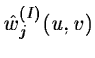=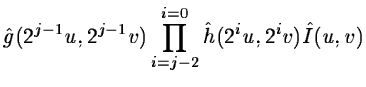=(14.106) =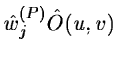where wj(P) are the wavelet coefficients of the PSF at the scale j. The wavelet coefficients of the image I are the product of convolution of object O by the wavelet coefficients of the PSF.

To deconvolve the image, we have to minimize for each scale j: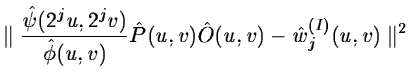(14.107)

and for the plane at the lower resolution: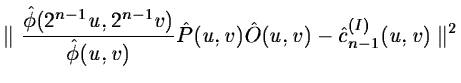(14.108)

n being the number of planes of the wavelet transform ((n-1) wavelet coefficient planes and one plane for the image at the lower resolution). The problem has not generally a unique solution, and we need to do a regularization . At each scale, we add the term: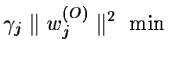(14.109)

This is a smoothness constraint. We want to have the minimum information in the restored object. From equations 14.107, 14.108, 14.109, we find:(14.110)

with:and:if the equation is well constrained, the object can be computed by a simple division of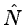by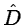. An iterative algorithm can be used to do this inversion if we want to add other constraints such as positivity. We have in fact a multiresolution Tikhonov's regularization. This method has the advantage to furnish a solution quickly, but optimal regularization parameters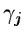cannot be found directly, and several tests are generally necessary before finding an acceptable solution. Hovewer, the method can be interesting if we need to deconvolve a big number of images with the same noise characteristics. In this case, parameters have to be determined only the first time. In a general way, we prefer to use one of the following iterative algorithms.Next: Regularization from significant structures Up: Deconvolution Previous: Regularization in the wavelet
Petra Nass
1999-06-15